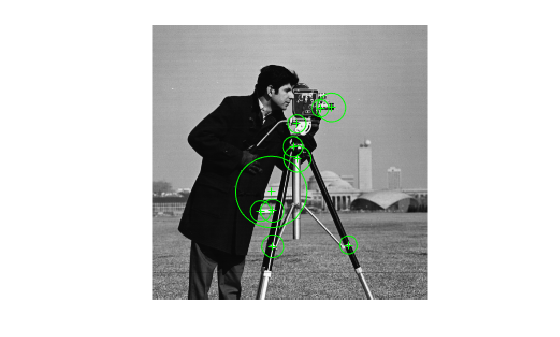# SIFTPoints

Object for storing SIFT interest points

## Description

The `SIFTPoints` object enables you to pass data between the `detectSIFTFeatures` and `extractFeatures` functions. You can also use it to manipulate and plot the data returned by these functions. You can use the object to fill interest points interactively.

## Creation

### Syntax

``points = SIFTPoints(location)``
``points = SIFTPoints(location,Name=Value)``

### Description

example

````points = SIFTPoints(location)` constructs a `SIFTPoints` object from an M-by-2 matrix of [x y] point coordinates in `location`. ```
````points = SIFTPoints(location,Name=Value)` sets properties using one or more name-value arguments. For example, `SIFTPoints(Metric=0.0)````

### Input Arguments

expand all

Point coordinates, specified as an M-by-2 matrix of [x y] point coordinates. M is the number of points.

## Properties

expand all

Scale at which the interest points are detected, specified as a value greater than `0`.

Strength of the detected feature, specified as a value in the range ```[0 1]```. The SIFT algorithm uses the contrast threshold to determine strong features.

Orientation of the detected feature, specified as an angle, in radians. The angle is measured counterclockwise from the X-axis with the origin specified by the `location` input. Do not set this property manually. Instead, use the call to `extractFeatures` to fill in this value. The `extractFeatures` function modifies the default value of `0.0`.The `Orientation` is mainly useful for visualization purposes.

Index of the Gaussian pyramid octave layer that the keypoint is extracted from, specified as an integer. An octave is a collection of difference-of-Gaussian pyramid layers, and is used for scale-space.

Layer within the octave from which the keypoint was extracted, specified as an integer.

## Object Functions

 `plot` Plot points `isempty` Determine if points object is empty `length` Number of stored points `selectStrongest` Select points with strongest metrics `size` Return size of points object `selectUniform` Select uniformly distributed subset of feature points

## Examples

collapse all

`I = imread("cameraman.tif");`

Detect SIFT features in the image.

`points = detectSIFTFeatures(I)`
```points = 274x1 SIFTPoints array with properties: Scale: [274x1 single] Orientation: [274x1 single] Octave: [274x1 int32] Layer: [274x1 int32] Location: [274x2 single] Metric: [274x1 single] Count: 274 ```

Display the location and scale of the 10 strongest points in the image.

```strongest = points.selectStrongest(10); imshow(I) hold on plot(strongest) ```Display the [x y] coordinates for the selected points.

`strongest.Location`
```ans = 10x2 single matrix 183.2607 205.7324 112.7613 206.8682 140.7289 96.1705 135.5473 125.4136 101.5411 174.0036 111.1929 156.2148 167.3374 77.7732 131.6280 114.0382 114.2321 48.5672 135.2517 92.6351 ```

Read an image into the workspace.

`I = imread("cameraman.tif");`

Detect SIFT features in the image.

`points = detectSIFTFeatures(I);`

Display the last 5 detected points.

```imshow(I) hold on plot(points(end-4:end))```## Tips

• Although `SIFTPoints` may hold many points, it is a scalar object. Therefore, `numel`(`SIFTPoints`) always returns `1`. This value may differ from `length`(`SIFTPoints`), which returns the true number of points held by the object.

• Properties can be specified as a scalar or a vector whose length matches the number of coordinates in `location`.

 Lowe, David G.. "Distinctive Image Features from Scale-Invariant Keypoints." Int. J. Comput. Vision 60 , no. 2 (2004): 91--110.